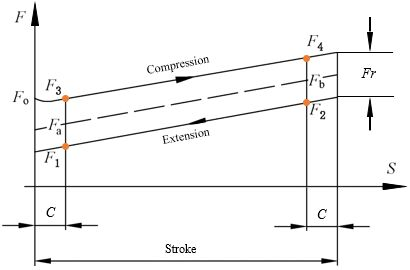# Explanation of gas strut forceForce explanations:
F0 = Breakaway force
F1 = Min. extension force
F2 = Max. extension force
F3 = Min. compression force
F4 = Max. compression force
Fa = Nominal force
Fr = Friction force

Method of measurement:
Before measurement, compress the gas strut to its entire stroke 2 or 3 times, then let the gas strut be fully extended.

a) Stroke ≤80mm, C=5mm
F1: Compress the gas strut 10mm, then extend it 5mm. Measure the force at this position.
F2: Compress the gas strut to its entire stroke, then extend it 5mm. Measure the force at this position.
F3: Compress the gas strut 5mm. Measure the force at this position.
F4: Compress the gas strut to the point 5mm from its stroke end. Measure the force at this position.

b) Stroke >80mm, C=10mm
F1: Compress the gas strut 20mm, then extend it 10mm. Measure the force at this position.
F2: Compress the gas strut to its entire stroke, then extend it 10mm. Measure the force at this position.
F3: Compress the gas strut 10mm. Measure the force at this position.
F4: Compress the gas strut to the point 10mm from its stroke end. Measure the force at this position.